## 21.6 The Slope of the implied Line of the Difference Series

In the past sections we examined the Approach of the F Series to its Limit algebraically. First we looked at the difference between consecutive members of the F Series. Then we examined the internal structure of the D Series for clues. In this section we will explore the geometry of the Approach of the F Series to its Limit. In so doing we will visually ‘see’ some of the concepts that we’ve explored algebraically.

### A. Difference Graphs for √2

#### Ordinary perspective

Below is a graph of the difference between our F Series for √2 and its limit, the √2 minus one. The X-axis represents the number of iterations while the Y-axis represents the difference between the F Series and its limit. We will call this series of differences the Difference Series. (Note that this is different from the F Series Difference that we explored above, which has no reference to the Limit.)Notice that our F Series is already within a thousandth of its limit after only 4 iterations . Visually the F Series has reached the Limit from this ‘ordinary’ perspective.

#### Difference Series from a logarithmic perspective

Now let us look at this same Difference Series from a logarithmic perspective. Below is a graph that will visually introduce you to some of our friends that we've only spoken about on an abstract level. Here we show how our Difference series for the square root of 2 approaches zero. Each bar represents how far the specified member of the Difference Series differs from zero. It is readily apparent that the odd functions are less than √2 while the even functions are greater than the √2. Furthermore, each successive element becomes closer and closer to √2 in a straight logarithmic manner. This reflects the geometric nature of the D Series. Notice the logarithmic scale of the y-axis. It is easy to see that the ratio of successive bars would have to be negative because the bars alternate from positive to negative.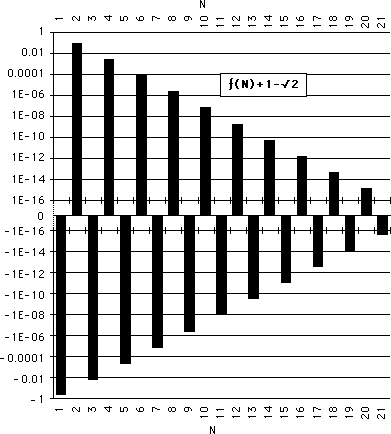Notice that our F series ratio never reaches its Limit from this logarithmic perspective, although it gets continuously closer. Further notice that the Difference between the F Series and its Limit alternates between negative and positive. Finally it seems that from this perspective that the Difference is actually linear.

#### Absolute value of the Difference Series

This linear relation is quite apparent when we graph the absolute difference of the same Differences between the F Series and its Limit. Each difference seems like it is a little less than 10 times closer to zeroThe notion we get is that with each iteration that the F Series approximation of the Root minus one gets linearly closer to its Limit from this logarithmic perspective. First it is a little above its Limit, and then it is a little closer but below its limit. And then it is a little closer still but above its Limit again. This pattern of a little above and then a little closer below and then a little closer above repeats itself, seemingly forever. The F Series gets closer and closer but never reaches its Limit.

#### How fast is the F Series approaching its Limit?

Of course when a mathematician sees a line, he wants to know what its slope is. This is because much information can be derived from that slope. For instance, now that we have a line with a slope, we have a velocity. Once we can determine the slope of this line we will have the velocity of the approach of successive members of the F Series to its Limit. We will then be able to answer the question: How fast is the F series approaching its Limit?

#### The Line and its Slope

Because of the importance of the computation of the slope of the implied line connecting the tops of all of our bars in the above graph, we will call this line, The Line. Similarly we will call the slope of this line, The Line, The Slope. See below.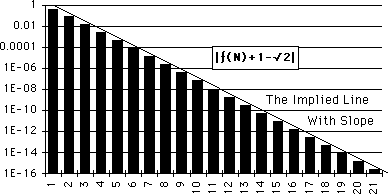The thrust of this section will be to find The Slope of The Line so that we can find The Equation for the Line. Hopefully the yield will be fruitful. Read on if you’re curious.

### B. The conjugates, x and x'

In order to discover the Slope, which will reveal the logarithmic linear velocity of the implied line in the above graph of the Difference Series, we need to first explore some more relations between the F Series and its inverse, the F’ Series. In doing so we will come in contact with some of the polarities of this iterative number family of Square Roots.

#### Basic relations of x to x'

Above we saw that x is the Limit of the F Series, ƒ(∞). Here we write it in a different fashion.We let x' equal the limit of the reciprocal function, ƒ'(∞), developed earlier in the Notebook. We then write it in another form. Note that x' is the conjugate of x.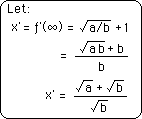These two quantities, x and x’, are to have an integral role in developing the properties of our Iterative Root Family. In fact these quantities will prove much more useful than the Root itself, as we shall see. The basic relations between x and x’ are to determine some crucial features of our system of rational roots, to be referred to as The System. Let us start with what we already know.

#### Difference and sum of x and x’

We remember that the difference between x and x’ is two. Below are some basic relations that follow from the definitions immediately above.Notice that the first relationship is merely the restating of the Difference Theorem B with the x-x’ notation. The second is their sum. The third shows that the ratio of their sum to their difference is the Root itself. While interesting these are merely curiosities compared to the ratio and product of conjugates that follow.

#### The divine ratio: x'/x = d

To find the ratio of x' to x, we merely simplify algebraically. This is to turn out to be a crucial ratio to our system.Eventually we will call this d, the divine ratio. It is called divine because it reveals so much about The System. We will explore this shortly.

### C. Product of Conjugates: x'*x = c = a/b - 1

#### The product: x*x' = c

The product of x and x’ is also to prove crucial to the structure of our system. It contributes to the understanding of the underlying polarity as well as contributing to the proofs.Equation 3 states what we already know, i.e. the two reciprocal functions both equal the rational square root. To find out the product of x and x' we multiply these two implied equations together, Equation 4. We then expand our equation, Equation 5. Remembering the fundamental relation between the function and its inversion, Eq. 6, we substitute for the difference of the function and its inverse, Eq. 7. We simplify, Eq. 8. Finally we write the left side of the equation in another form. This yields the product of x and x', Eq. 9.

#### Letting c = Root Number minus one

The primacy of this value is so great that we call it c, the constant. Restating we will let the product of the conjugates, x' and x, equal c, which equals (a-b)/b, which equals the Root Number, a/b, minus one. Shown below.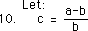Again for our System ‘c’ will prove a more fundamental constant than the Root Number itself, a/b. Just as the Root is superseded by the F Series Limit, ‘x’, so is the Root Number superseded by ‘c’. Just as x equals the Root minus one, so does c equal the Root Number minus one. There is a reason, which we will eventually discover. But it is not time yet.

#### Simplification of the Infinite Continued Fraction

Let us see how the constant c simplifies our infinite continued fraction. Rewriting Eq. 9 with the ‘c’ notation,Step 12 is the expression for the infinite continued fraction for the square root of any rational number that we derived previously. We can write the same equation with the constant c, yielding a simplified form of the same equation, step 13.#### Simplified F Series Theorem

Further the F Series Theorem for ƒ(N) could also be written in simplified form.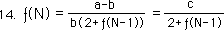This form with the constant c is one we will use for the F Series. The a and b constants will be used primarily for the D Series.

#### The finite manifestation of c

We find another appearance of this same constant, c, in the following manifestation. This relation will prove crucial in a proof to come. It is the essential link between the finite and infinite.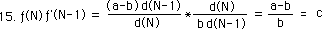#### Connection between Finite and Infinite, Theorems, 5th, 6th and 7th

We now have the fifth, sixth and seventh Theorems which relate the finite and infinite manifestations of the F Series and its inversion. These are listed below.These are all based around the givens that x is the limit of the F Series and that x’ is the limit of the F’ Series. The F Series and F’ Series were defined in the 1st and 4th Theorems. Theorem 5 is from equation 9 above, while Theorem 6 is from Equation 15. Theorem 7 is merely the combination of Theorems 5 and 6.

#### Obvious, not really so obvious

On first glance Theorem 7 seems obvious because x and x’ are the Limits of F and F’. However limits imply approximations on the finite level. In other words, the F Series only ≈ its limit x and the F’ series only ≈ its limit x’. Individually the Series only approximates the Limits, while as a product the Series equal the Limits. Further their products both equal c, the Root Number minus one. A tightly crystalline system.

### D. ‘d’, Ratio of conjugates: x'/x = d or the Divine Ratio

Let us now examine the ratio of conjugates. This will also prove to be a very fundamental relation of Root Family System. We will call it d.#### Thrust of section to find The Slope of The Line

Remember that the thrust of this section is to find The Slope of the implied Line of the Difference Series. This Line with its Slope was shown in the graphs that we started this section with. Remember that this Slope will tell us how quickly our F Series approaches its Limit, the Square Root of any Rational Number minus One. Now we have the tools to solve our problem.

#### The ratio of the consecutive differences & The Slope

From the graph it seems that the ratio between consecutive members of the Difference Series is constant. Therefore let us look at this ratio. The algebraic expression for the ratio of these differences is shown below. The denominator is the Nth element of the Difference Series, which equals the difference between the F Series and its Limit. The numerator is the preceding element of the Difference Series, the N-1 element. This ratio is what we are looking for.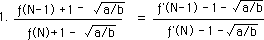We can write the same ratio in terms of the reciprocal functions, on the right. This is because of the Difference Theorem where ƒ’ - ƒ = 2. Some simple algebra yields ƒ + 1 = ƒ’ - 1. Using this substitution on the left side of the equation yields the right side.

#### ƒ - x = ƒ’ - x’

Let us remember the notational simplification that we adopted, where x and x' are conjugates. Remember "x" is the limit of our F series, ƒ(∞), while x' is the limit of our inverse series F’ or ƒ'(∞).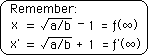Returning to equation 1, we substitute our "x” s and "x'" s into the appropriate sides of the equation.Note that the last fraction contains the prime expression on the top, while containing the regular functions on the bottom. Mix and match. This is because ƒ - x = ƒ’ - x’. This simple proof is based on the application of both Difference Theorems. ƒ - x = (ƒ’- 2) - (x’ - 2) = ƒ’ - x’.

#### Applying the mix of finite and infinite, Theorems 5->7

Remember the finite and infinite expression for c from the previous section, Theorems 5, 6, & 7..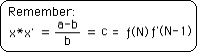We equate the first and last parts of the above equation and solve for ƒ(N).Substituting for ƒ(N) in equation 2. We then solve and simplify algebraically.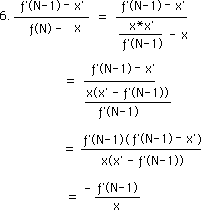#### The ratio of the consecutive differences from the square root = -d

The limit of ƒ'(N-1) as N->∞ is x'. Hence the above expression becomes -x'/x as N->∞.This then is the limit of the ratio of consecutive differences as N->∞. It is the negative of the Divine Ratio of conjugates.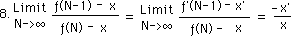Restating the same result in terms of our rational number, a/b, gives us our desired result. This has been confirmed experimentally and is shown in the appendices.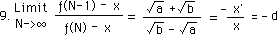### E. The Implications of the Divine Ratio and its Graph

Because that's the type of group we are, we will call x'/x, the Divine Ratio. This is because this ratio speaks volumes about our F Series and is easily computable.

#### Implications: Bracketing, a little above and below

What does this all mean? First when a > b, i.e. a/b > 1, our ratio is always negative because √b-√a in the denominator will always be negative. This means that consecutive differences will be of different signs. Hence although ƒ(N) -> x as N->∞, if ƒ(N) > x then ƒ(N-1) < x, in order that ƒ(N)-x and ƒ(N-1)-x be of different signs. We see from this that the square roots are not approached from a single direction but are instead bracketed, i.e., first a little over then a little under, steadily approaching the goal. But never reaching it. This is quite different from the repeating decimal, which always approaches the fraction from below.

#### Divine Ratio, how quickly the F Series approaches the Limit

The Divine Ratio tells how quickly our F Series approaches its Limit, i.e. the square root of any rational number minus one. For instance when a/b = 2 each consecutive ratio is approximately 6 times closer to the √2 than the ratio before. This has some interesting implications. First we see that the ratio approaches √2 steadily and rapidly. The 20th ratio is ≈ 6^20 closer to √2 than the first ratio. Practically speaking the ratios above 20 exceed the accuracy of most home computers.

#### F Series for √2 independent of Seed

The initial points of the iterative series are called ‘seeds’. We let the ‘seed’ of our F Series equal zero. However the results above imply that these beginning points have virtually no influence upon the result. The 20th iteration of the F Series for √2 will be ≈ 6^20 closer to √2 than the first iteration, no matter which seed starts the iteration process. If our first ratio were 1000, the 20th iteration would still be ≈ 6^20 closer to √2 than the first iteration. Thus the initial seed for this F Series only influences how quickly the series reaches its Limit, its Destination, but has no influence upon the Destination itself.

#### The pattern of the F&D Series transcends the Seed

The point to be made is that the pattern of the F&D Series for the square root of two transcends the Seed in importance. The pattern of the F&D Series is more important than any starting points in determining the Limit. No matter what is chosen for the first member of the F Series, the pattern eventually transforms the seed into the desired square root. Hence changing the initial input changes nothing except the speed at which a more accurate √2 is reached. The initial input has nothing to do with the inevitable conclusion.

#### Each D series approaches a different limit independent of starting point

Only by changing the parameters of the F&D Series will the Limit be changed. Changing the parameters will immediately change the Limit. Each F&D Series leads to a specific conclusion independent of what went before. Hence in the F&D Series System the starting points, or seeds, have virtually nothing to do with the conclusion. The only thing that matters is the pattern. If the pattern is changed the result is changed. In some ways the D series is the underlying pattern of the designated square root. Back to trees and seeds: Perhaps the acorn is programmed with some type of similar patterning which is also independent of input. No matter what input is given to the seed, an oak pattern is generated which eventually yields an oak tree.

#### The Graph

Let us look at a graph of the function for the Divine Ratio to understand some of its implications.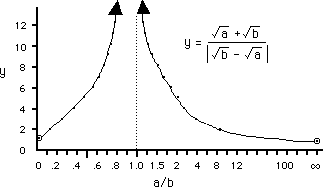#### The Symmetry

Our equation is symmetrical for a and b. Hence the area between 0 and 1 is equivalent to the area between 1 to ∞, i.e. they both behave identically, symmetrical about the axis at one. The numbers and their inverse behave identically in terms of their approach to the limit. They approach at the same speed although they approach different limits. Keeping this symmetry in mind, we continue our discussion.

#### The closer to one, the quicker it approaches its limit

We've only spoken of the series associated with the √2. We mentioned that it approaches the √2 at a rate of approximately 6. Let us look at some other points. As b approaches a, the denominator of our Divine Ratio approaches zero and its value explodes. See graph when a/b =1. This means that the closer to one that a/b is, the more quickly the F&D Series approach the Limit, i.e. the Root minus one. When a = b or a/b = 1 then the ratio of the denominator series is immediately the root, i.e. √1 = 1, instantly and always. The closer to one the quicker it approaches the root. On the lower side of one, at 1/9, our series approaches at a rate of 2, i.e. our 20th ratio will be 2^20 closer than will be the first. We have a similar condition on the other end. When a/b = 9, the ratios approach at a rate of 2. This is still quick. As pointed out 2^20 is huge. This means that the difference between each consecutive ratio and the root is half the one before. Hence between 1/9 and 9, our ratios approach the root at a rate of 2 or more. Hence the starting points still have very little influence.

#### The further from one the slower the approach

Conversely, the further from one that our Root Number, a/b is, the slower the F&D Series approaches their Limit. Below 1/9, as a/b approaches zero, the rate approaches one. Above 9, as a/b approaches ∞, the rate also approaches one. Above 9 or below 1/9 the approach becomes slower and slower. As a/b approaches infinity or zero, the rate approaches one. The closer to one the rate is, the more at a standstill our ratios are. This increases the significance of the starting points because the rate of approach to the limit is so slow.

#### The importance of Starting Points at the Edges and the Stability of the Pattern in the middle

The slower the approach to the square root, the more importance the starting points has. Thus the pattern is everything in the in-between areas while the pattern assumes less and less significance on the edges. Vice versa the starting points have virtually no influence in the in-between areas while they assume great significance on the edges.